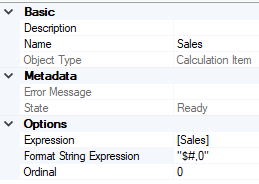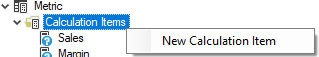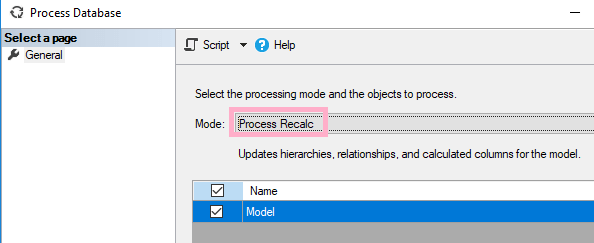I have been reading some great blog posts from SQLBI.COM on using and implementing Calculation Groups. What I did find is that currently there is not step by step process on how to get this working. This is what I will do in my blog post below.

As it stands today 29 April 2020 you can currently use calculation groups where the Analysis Services compatibility level is higher than 1470. What this means is that it is available in Power BI Pro & Premium, Azure Analysis Services and SQL Server Analysis Services 2019.

Not only that but listening to webinars by Daniel Otykier and Christian Wade they both mentioned that calculation groups will be coming to Power BI Desktop in a future release.

Here are the steps below to implement a calculation group where it allows a user to dynamically switch between the metrics. Not only that it also allows me to format the strings of each of the measures separately. What this means is that when the measure is displayed it will be correctly formatted. Unlike in the past where I had to use the FORMAT expression which caused the measure to be a STRING.

NOTE: All the steps below use Tabular Editor

## Example Measures

In my working example I am using data from the sample database Worldwide Importers.

I have created the following measures, which will be used in the steps below to create the Calculation Group

Measures:

`Sales = SUM ( 'Order'[Total Including Tax] )`
`Orders = COUNTROWS ( 'Order' )`
`Avg Order Size = DIVIDE ( [Sales], [Orders] )`
`Unit Cost = SUM ( 'Order'[Unit Price] )`
`Margin = DIVIDE ( [Sales], [Unit Cost]`

## Creating Metric Calculation Group

The Metric Calculation Group is used where I can put all the metrics that I want users to be able to use to dynamically change what they are viewing.

For example, I want to users to be able to change measures between Sales and Margin using a Slicer from a table.

Below shows how to do this with multiple measures

• Right click and select Create New, Calculation Group
•• I then gave it the name of Metric
•• Next, I renamed the column name to be called Metric
•• You will notice currently that there are NO Calculation Items
• A very important step is to set the Precedence for the Calculation Group
• NOTE: The higher the precedence will always be executed before the lower ones.
• For the Metric calculation group, I gave it a precedence of 10, because I want my next calculation group Currency Symbol to be run first and must have a precedence of 30
• Following on from this I then needed to create my Calculation Item (This is where the magic happens and I can define not only the expression for the calculated item, but also the Format String Expression
• I right clicked on Calculation Items and selected New Calculation Item
•• I have it the name Sales
•• Next, I made the expression the same as the name (This is so that it can be used dynamically later)
•• Next, I put in the Format String Expression which is a DAX expression with the following for Avg Bet Size
• “#,0”
• NOTE:
What I did learn is that the Format String Expression is actually being evaluated as a DAX expression. In order for the format to be recognized you have to treat it as you would with any DAX expression where you want it to be seen as text and wrap it in the double quotes as with my example above #,0
• And this is what my Calculation Item looks like for Avg Bet Size
•• I then created my additional Metics by right clicking on Calculation Items and clicking on New Calculation Item
•• I configured all my calculation tiems below with the name, expression and Format String Expression
 Name Expression Format String Expression Sales [Sales] “#,0” Margin [Margin] “#,##0.00 %” Orders [Orders] “#,##0” Avg Order Size [Avg Order Size] “\$ #,##0.00” Unit Cost [Unit Cost] “#,##0”
• The final step was to either set the Ordinal (The order in which they are displayed) or drag and drop them into the order as I did below
•• Now I have completed my Metric Calculation Group
• Because I am doing this using Power BI Premium, I deployed the changes to my Power BI Premium dataset.
• And in order to get the calculation group working I had to do a process recalc on the database as shown below.
•• I then connected to my dataset and created the following table below
•• As you can see above each of the values from my Metric table has got a different format string.
• What I can now also do is to create a Slicer which is based off my Metric table
•• I can then select 2 measures and show them in a table
•• Now that is very cool and something, we all have been asking for a long time and will make reports that much better and interactive for report consumers.

## Current Visuals that support Calculation Groups

I consider myself very fortunate to be able to get answers from the Power BI team and in this instance, I asked them which visuals are currently supported by the calculation groups, which are listed below

• Table
• Matrix
• Card
• Multi row card

The good news is that the other visuals are being worked on will be supported in a future release!

## Summary

In this blog post I have shown you how I created a calculation group, create the calculation items, configure the format strings and then how to use it in a Power BI report.

I do hope that you found this helpful.

As I say every time thanks for reading, and if you got any comments or suggestions please leave them in the comments section below.